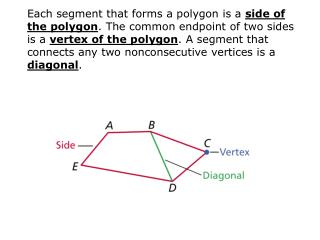DownloadDownload PresentationTriangle

# Triangle

Télécharger la présentation## Triangle

- - - - - - - - - - - - - - - - - - - - - - - - - - - E N D - - - - - - - - - - - - - - - - - - - - - - - - - - -
##### Presentation Transcript

1. Each segment that forms a polygon is a side of the polygon. The common endpoint of two sides is a vertex of the polygon. A segment that connects any two nonconsecutive vertices is a diagonal.

2. You can name a polygon by the number of its sides. The table shows the names of some common polygons. 3 Triangle 8 Octagon 4 9 Nonagon Quadrilateral 5 Pentagon 10 Decagon 6 12 Hexagon Dodecagon 7 n Heptagon N-gon

3. Remember! A polygon is a closed plane figure formed by three or more segments that intersect only at their endpoints. Example 1 Tell whether the figure is a polygon. If it is a polygon, name it by the number of sides. polygon, hexagon polygon, heptagon not a polygon polygon, nonagon not a polygon

4. All the sides are congruent in an equilateral polygon. All the angles are congruent in an equiangular polygon. A regular polygonis one that is both equilateral and equiangular. If a polygon is not regular, it is called irregular. A polygon is concave if any part of a diagonal contains points in the exterior of the polygon. If no diagonal contains points in the exterior, then the polygon is convex. A regular polygon is always convex.

5. Example 2: Tell whether the polygon is regular or irregular. Tell whether it is concave or convex. irregular, convex regular, convex regular, convex

6. In each convex polygon, the number of triangles formed is two less than the number of sides n. So the sum of the angle measures of all these triangles is (n — 2)180°. Example 3A: Find the sum of the interior angle measures of a convex heptagon. (n – 2)180° Polygon  Sum Thm. (7 – 2)180° A heptagon has 7 sides, so substitute 7 for n. 900° Simplify.

7. Find the measure of each interior angle of a regular 16-gon. Example 3B: Step 1 Find the sum of the interior angle measures. (n – 2)180° Polygon  Sum Thm. Substitute 16 for n and simplify. (16 – 2)180° = 2520° Step 2 Find the measure of one interior angle. The int. s are , so divide by 16.

8. Find the measure of each interior angle of pentagon ABCDE. Example 3C: (5 – 2)180° = 540° Polygon  Sum Thm. Polygon  Sum Thm. mA + mB + mC + mD + mE = 540° 35c + 18c+ 32c+ 32c+ 18c= 540 Substitute. 135c= 540 Combine like terms. c= 4 Divide both sides by 135. mA = 35(4°)= 140° mB = mE = 18(4°)= 72° mC = mD = 32(4°)= 128°

9. Remember! An exterior angle is formed by one side of a polygon and the extension of a consecutive side. In the polygons below, an exterior angle has been measured at each vertex. Notice that in each case, the sum of the exterior angle measures is 360°.

10. measure of one ext.  = Example 4A: Finding Interior Angle Measures and Sums in Polygons Find the measure of each exterior angle of a regular 20-gon. A 20-gon has 20 sides and 20 vertices. sum of ext. s = 360°. Polygon  Sum Thm. A regular 20-gon has 20  ext. s, so divide the sum by 20. The measure of each exterior angle of a regular 20-gon is 18°.

11. Example 4B: Finding Interior Angle Measures and Sums in Polygons Find the value of b in polygon FGHJKL. Polygon Ext.  Sum Thm. 15b° + 18b° + 33b° + 16b° + 10b° + 28b°= 360° 120b= 360 Combine like terms. b= 3 Divide both sides by 120.

12. Example 5: Art Application Ann is making paper stars for party decorations. What is the measure of 1? 1 is an exterior angle of a regular pentagon. By the Polygon Exterior Angle Sum Theorem, the sum of the exterior angles measures is 360°. A regular pentagon has 5  ext. , so divide the sum by 5.